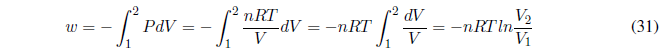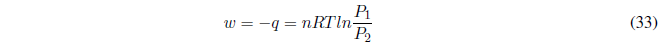During this process the temperature is kept constant. Since the internal energy of ideal gases only depends on temperature: $\Delta U=0$ and $\Delta H=0$.

The first principle tells us: $\Delta U=q+w\;\;\rightarrow q=-w$ . In this process, the heat exchanged is equal to the work done. We calculate the work done by the gas using the equation of state.It can be put as a function of the initial and final state pressures using Boyle's law,Substituting: